Скачать презентацию Shopping with Fried Worms Pay to the Next

8b5f471f03693181b9f12556badd30ca.ppt

• Количество слайдов: 26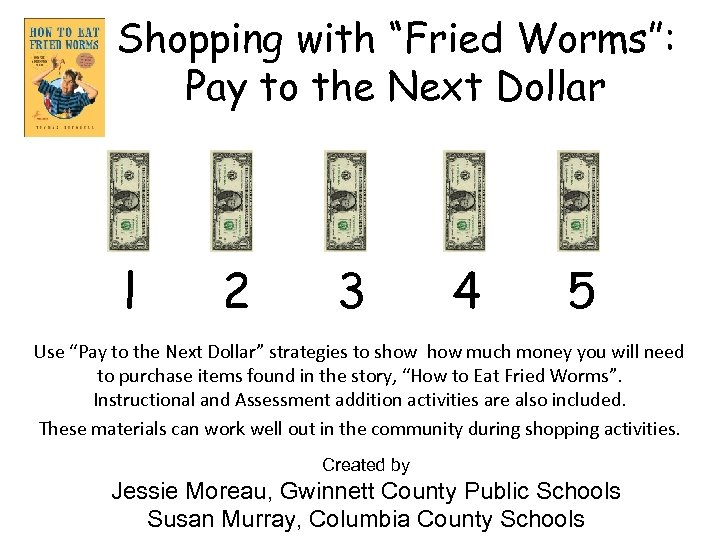Shopping with “Fried Worms”: Pay to the Next Dollar l 2 3 4 5 Use “Pay to the Next Dollar” strategies to show much money you will need to purchase items found in the story, “How to Eat Fried Worms”. Instructional and Assessment addition activities are also included. These materials can work well out in the community during shopping activities. Created by Jessie Moreau, Gwinnett County Public Schools Susan Murray, Columbia County Schools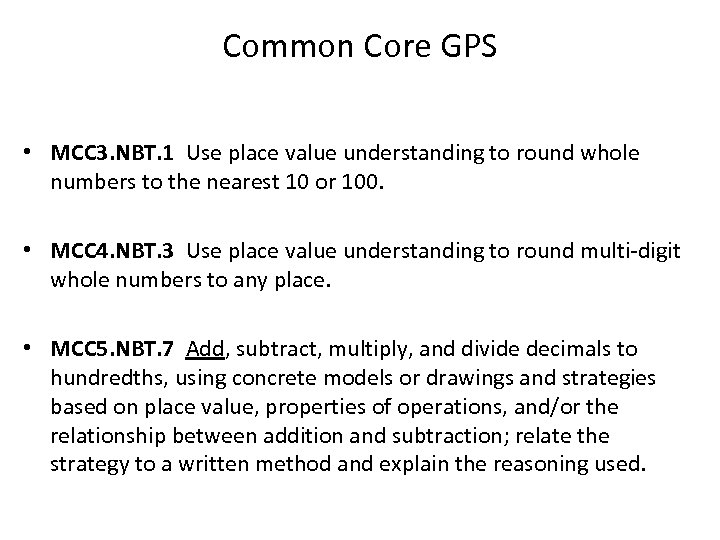Common Core GPS • MCC 3. NBT. 1 Use place value understanding to round whole numbers to the nearest 10 or 100. • MCC 4. NBT. 3 Use place value understanding to round multi‐digit whole numbers to any place. • MCC 5. NBT. 7 Add, subtract, multiply, and divide decimals to hundredths, using concrete models or drawings and strategies based on place value, properties of operations, and/or the relationship between addition and subtraction; relate the strategy to a written method and explain the reasoning used.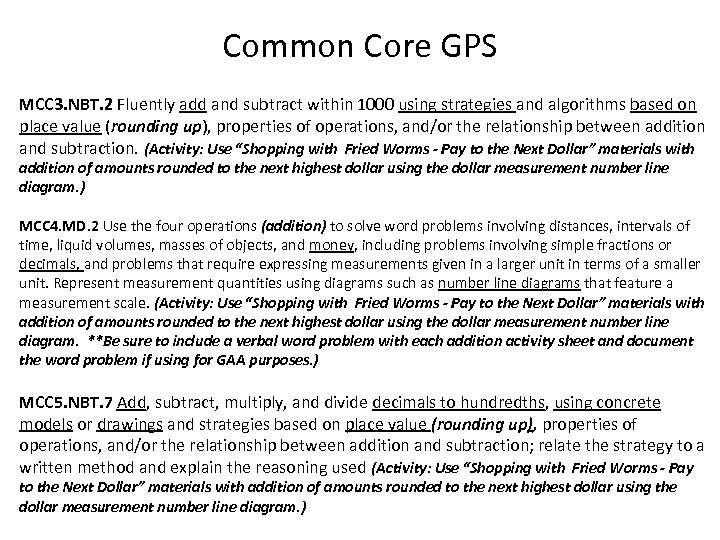Common Core GPS MCC 3. NBT. 2 Fluently add and subtract within 1000 using strategies and algorithms based on place value (rounding up), properties of operations, and/or the relationship between addition and subtraction. (Activity: Use “Shopping with Fried Worms - Pay to the Next Dollar” materials with addition of amounts rounded to the next highest dollar using the dollar measurement number line diagram. ) MCC 4. MD. 2 Use the four operations (addition) to solve word problems involving distances, intervals of time, liquid volumes, masses of objects, and money, including problems involving simple fractions or decimals, and problems that require expressing measurements given in a larger unit in terms of a smaller unit. Represent measurement quantities using diagrams such as number line diagrams that feature a measurement scale. (Activity: Use “Shopping with Fried Worms - Pay to the Next Dollar” materials with addition of amounts rounded to the next highest dollar using the dollar measurement number line diagram. **Be sure to include a verbal word problem with each addition activity sheet and document the word problem if using for GAA purposes. ) MCC 5. NBT. 7 Add, subtract, multiply, and divide decimals to hundredths, using concrete models or drawings and strategies based on place value (rounding up), properties of operations, and/or the relationship between addition and subtraction; relate the strategy to a written method and explain the reasoning used (Activity: Use “Shopping with Fried Worms - Pay to the Next Dollar” materials with addition of amounts rounded to the next highest dollar using the dollar measurement number line diagram. )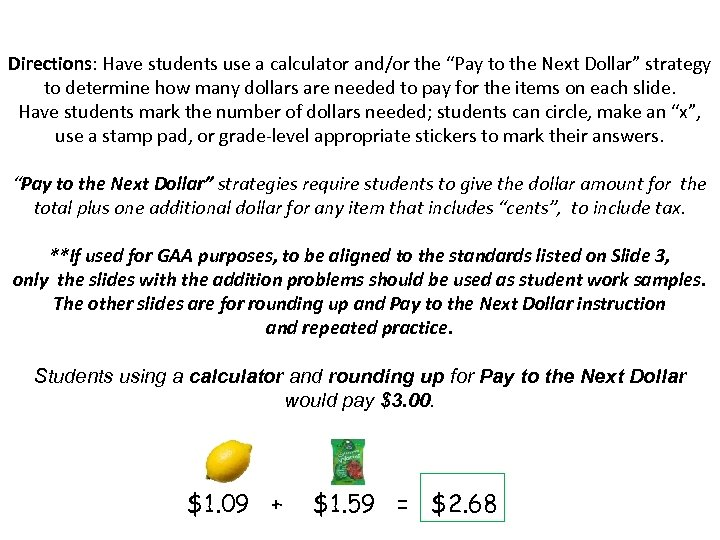Directions: Have students use a calculator and/or the “Pay to the Next Dollar” strategy to determine how many dollars are needed to pay for the items on each slide. Have students mark the number of dollars needed; students can circle, make an “x”, use a stamp pad, or grade‐level appropriate stickers to mark their answers. “Pay to the Next Dollar” strategies require students to give the dollar amount for the total plus one additional dollar for any item that includes “cents”, to include tax. **If used for GAA purposes, to be aligned to the standards listed on Slide 3, only the slides with the addition problems should be used as student work samples. The other slides are for rounding up and Pay to the Next Dollar instruction and repeated practice. Students using a calculator and rounding up for Pay to the Next Dollar would pay \$3. 00. \$1. 09 + \$1. 59 = \$2. 68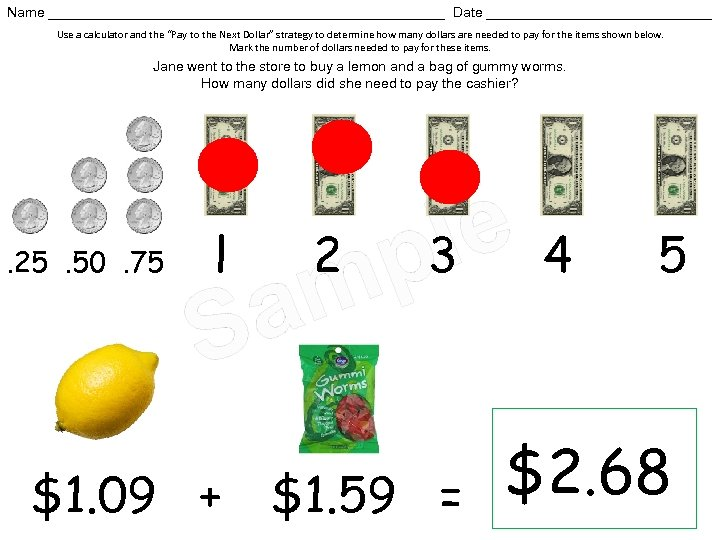Name __________________________ Date _______________ Use a calculator and the “Pay to the Next Dollar” strategy to determine how many dollars are needed to pay for the items shown below. Mark the number of dollars needed to pay for these items. Jane went to the store to buy a lemon and a bag of gummy worms. How many dollars did she need to pay the cashier? . 25. 50. 75 l 2 m a S \$1. 09 + le 4 p 3 \$1. 59 = 5 \$2. 68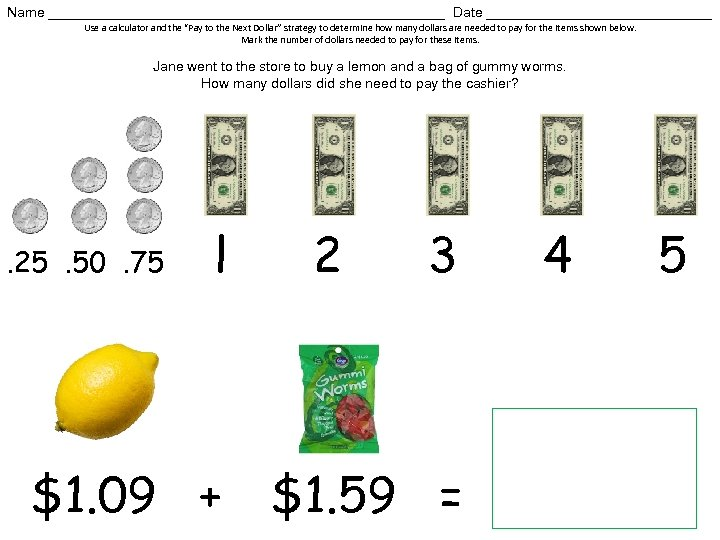Name __________________________ Date _______________ Use a calculator and the “Pay to the Next Dollar” strategy to determine how many dollars are needed to pay for the items shown below. Mark the number of dollars needed to pay for these items. Jane went to the store to buy a lemon and a bag of gummy worms. How many dollars did she need to pay the cashier? . 25. 50. 75 l \$1. 09 + 2 3 \$1. 59 = 4 5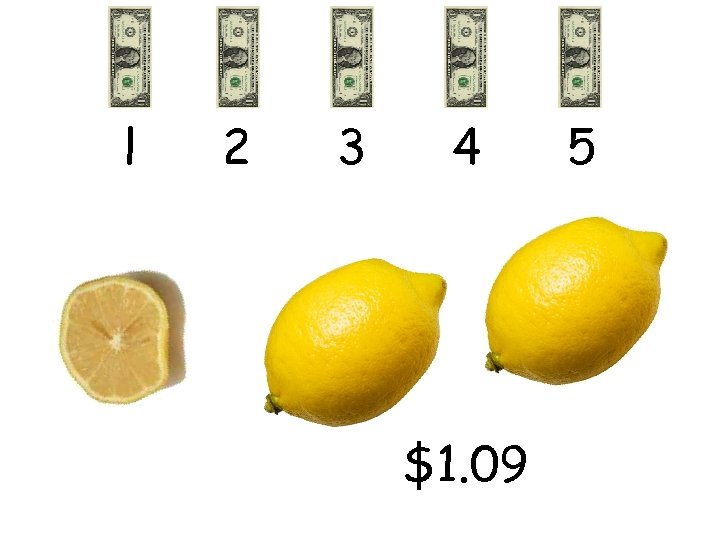l 2 3 4 \$1. 09 5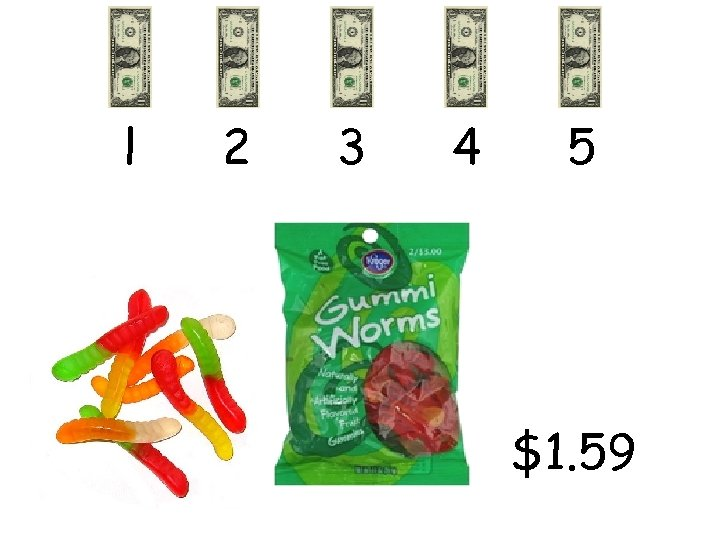l 2 3 4 5 \$1. 59Name __________________________ Date _______________ Use a calculator and the “Pay to the Next Dollar” strategy to determine how many dollars are needed to pay for the items shown below. Mark the number of dollars needed to pay for these items. Jane went to the store to buy a lemon and a bag of gummy worms. How many dollars did she need to pay the cashier? . 25. 50. 75 l \$1. 09 + 2 3 \$1. 59 = 4 5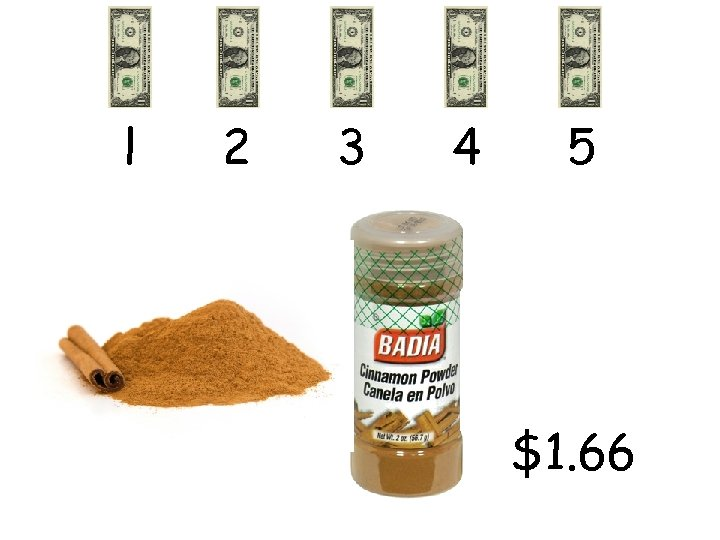l 2 3 4 5 \$1. 66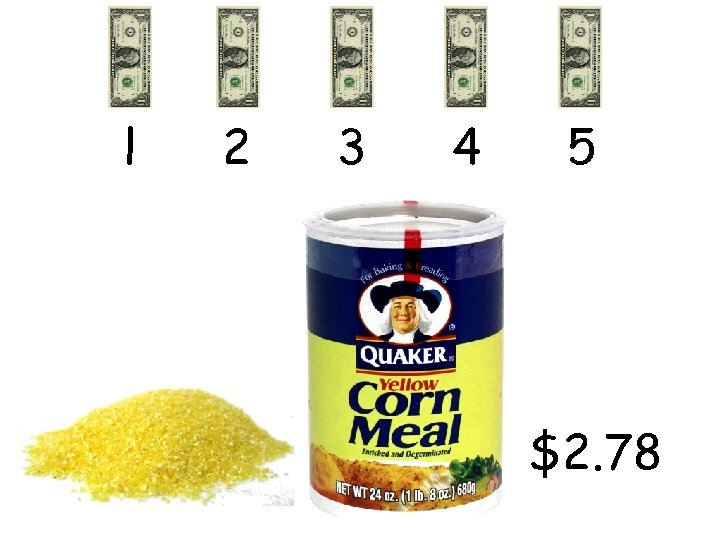l 2 3 4 5 \$2. 78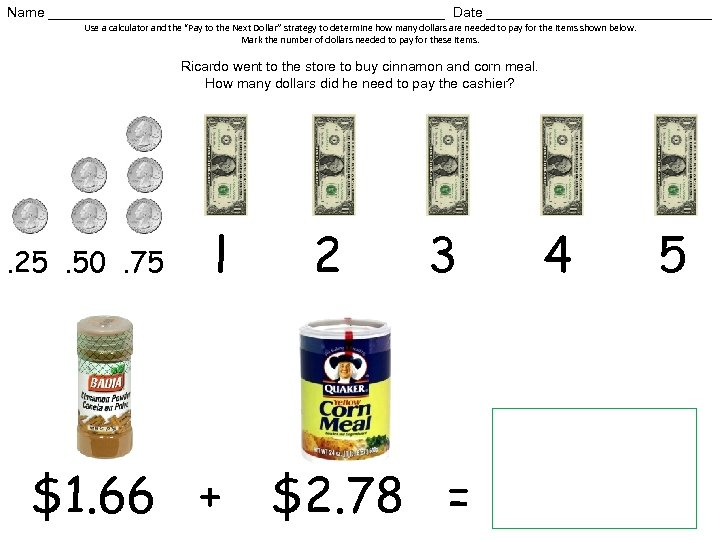Name __________________________ Date _______________ Use a calculator and the “Pay to the Next Dollar” strategy to determine how many dollars are needed to pay for the items shown below. Mark the number of dollars needed to pay for these items. Ricardo went to the store to buy cinnamon and corn meal. How many dollars did he need to pay the cashier? . 25. 50. 75 l \$1. 66 + 2 3 \$2. 78 = 4 5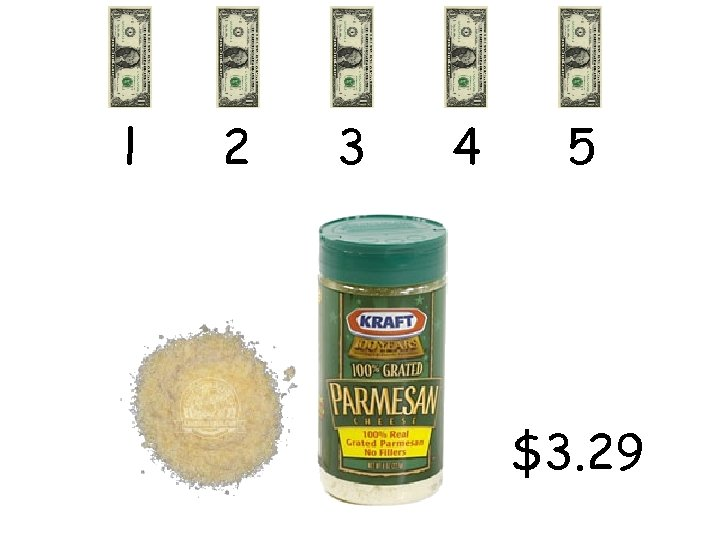l 2 3 4 5 \$3. 29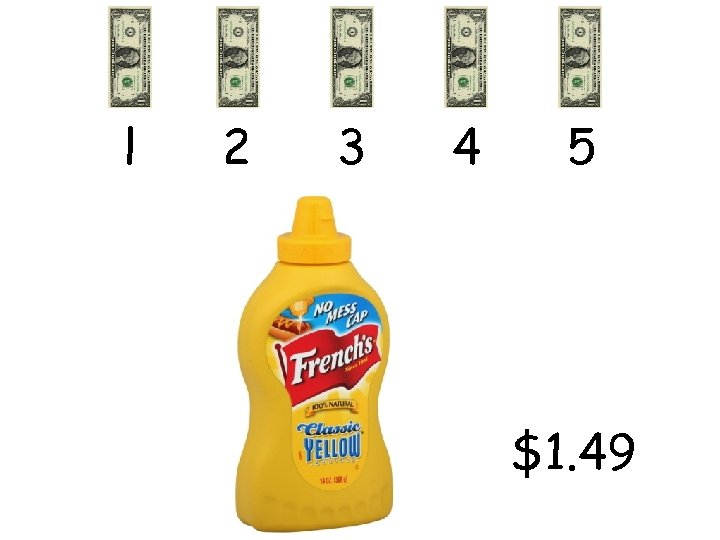l 2 3 4 5 \$1. 49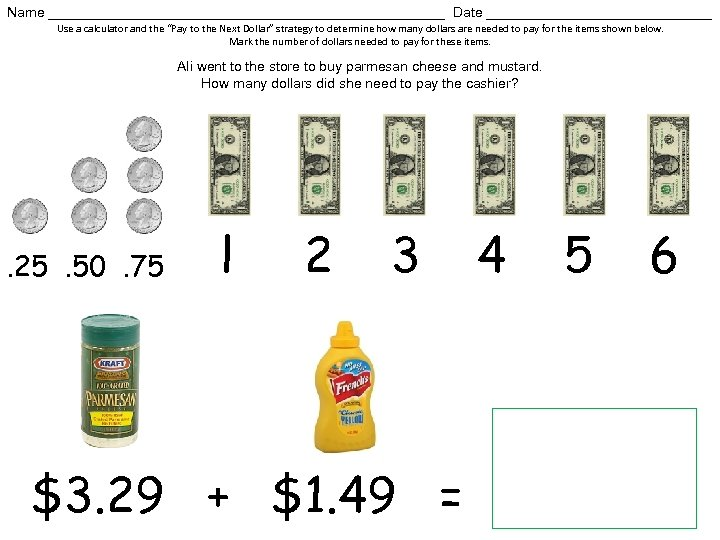Name __________________________ Date _______________ Use a calculator and the “Pay to the Next Dollar” strategy to determine how many dollars are needed to pay for the items shown below. Mark the number of dollars needed to pay for these items. Ali went to the store to buy parmesan cheese and mustard. How many dollars did she need to pay the cashier? . 25. 50. 75 l 2 3 \$3. 29 + \$1. 49 = 4 5 6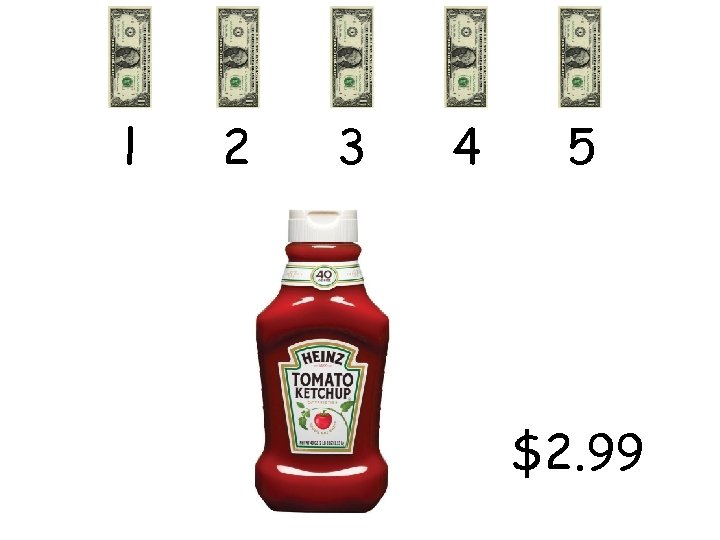l 2 3 4 5 \$2. 99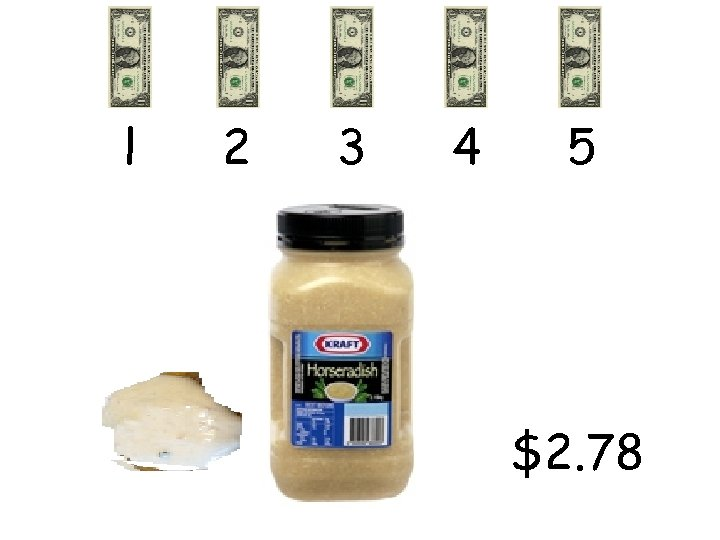l 2 3 4 5 \$2. 78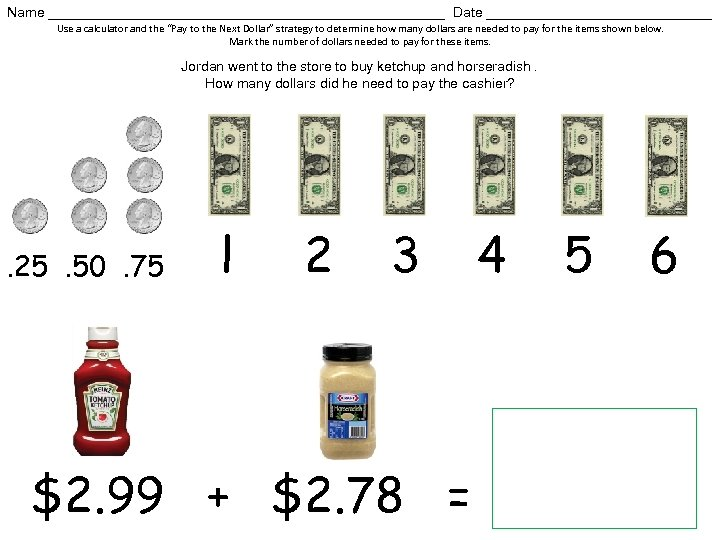Name __________________________ Date _______________ Use a calculator and the “Pay to the Next Dollar” strategy to determine how many dollars are needed to pay for the items shown below. Mark the number of dollars needed to pay for these items. Jordan went to the store to buy ketchup and horseradish. How many dollars did he need to pay the cashier? . 25. 50. 75 l 2 3 \$2. 99 + \$2. 78 = 4 5 6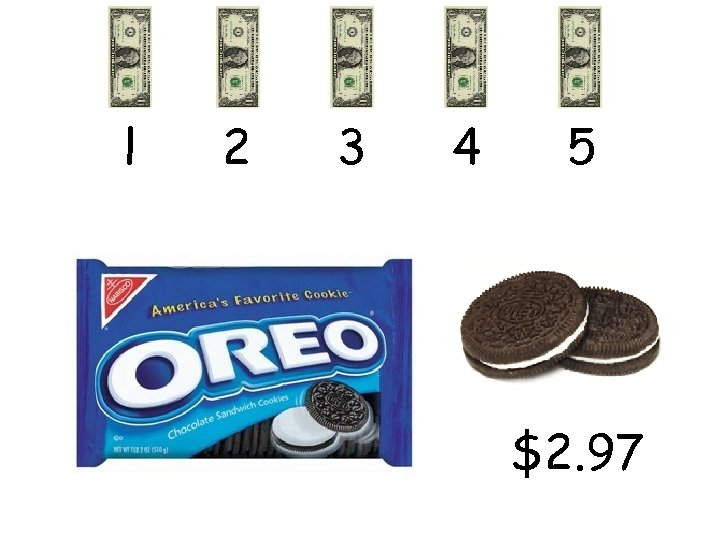l 2 3 4 5 \$2. 97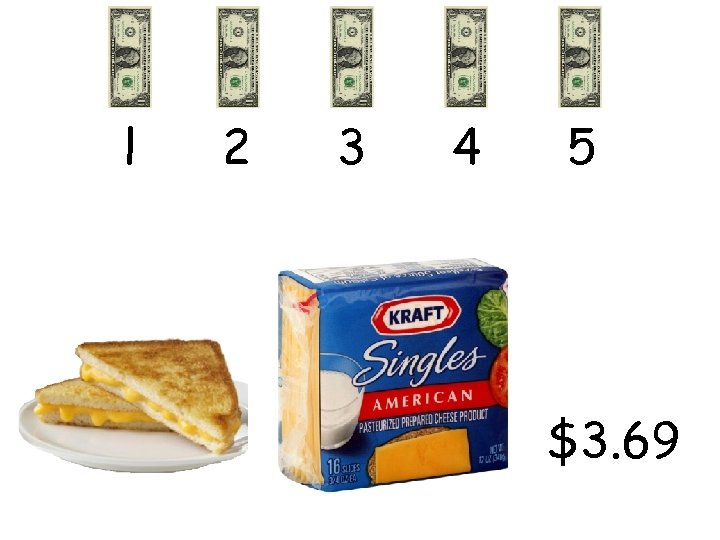l 2 3 4 5 \$3. 69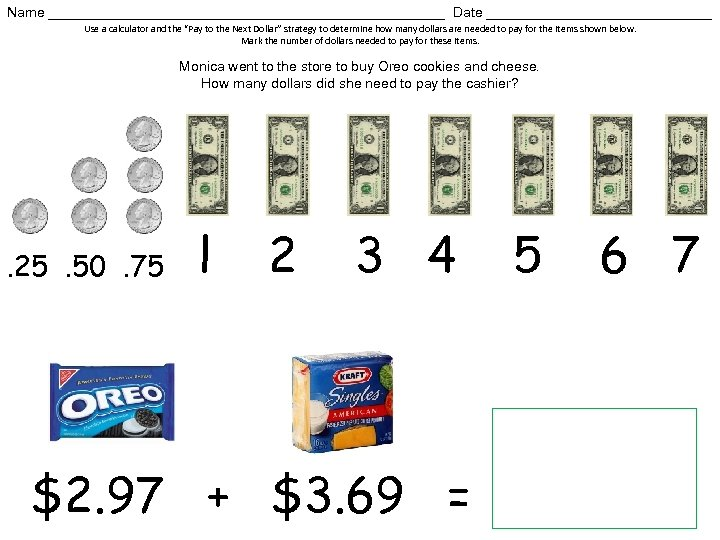Name __________________________ Date _______________ Use a calculator and the “Pay to the Next Dollar” strategy to determine how many dollars are needed to pay for the items shown below. Mark the number of dollars needed to pay for these items. Monica went to the store to buy Oreo cookies and cheese. How many dollars did she need to pay the cashier? . 25. 50. 75 l 2 3 4 \$2. 97 + \$3. 69 = 5 6 7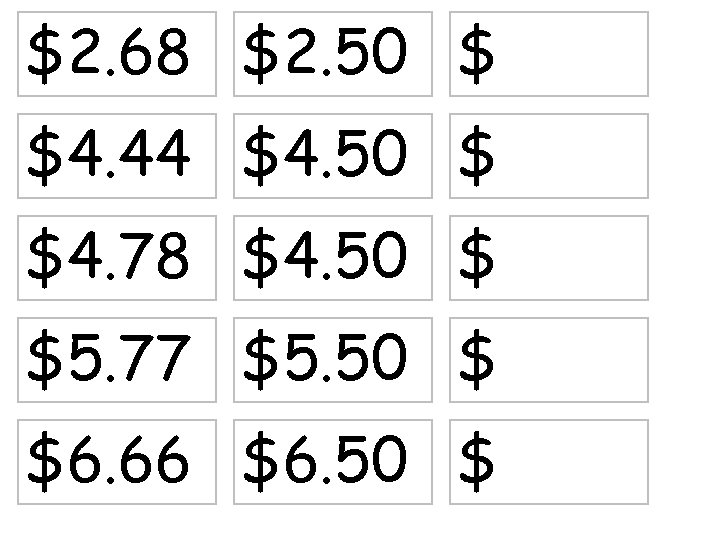\$2. 68 \$2. 50 \$ \$4. 44 \$4. 50 \$ \$4. 78 \$4. 50 \$ \$5. 77 \$5. 50 \$ \$6. 66 \$6. 50 \$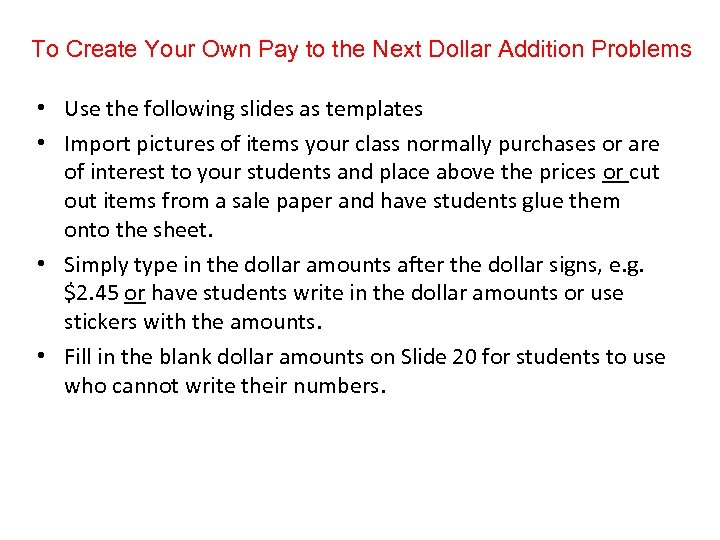To Create Your Own Pay to the Next Dollar Addition Problems • Use the following slides as templates • Import pictures of items your class normally purchases or are of interest to your students and place above the prices or cut out items from a sale paper and have students glue them onto the sheet. • Simply type in the dollar amounts after the dollar signs, e. g. \$2. 45 or have students write in the dollar amounts or use stickers with the amounts. • Fill in the blank dollar amounts on Slide 20 for students to use who cannot write their numbers.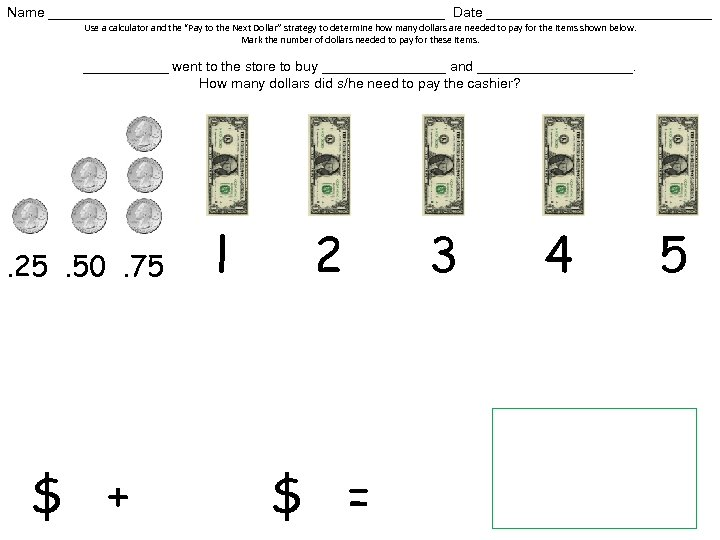Name __________________________ Date _______________ Use a calculator and the “Pay to the Next Dollar” strategy to determine how many dollars are needed to pay for the items shown below. Mark the number of dollars needed to pay for these items. ______ went to the store to buy ________ and __________. How many dollars did s/he need to pay the cashier? . 25. 50. 75 \$ + l 2 \$ = 3 4 5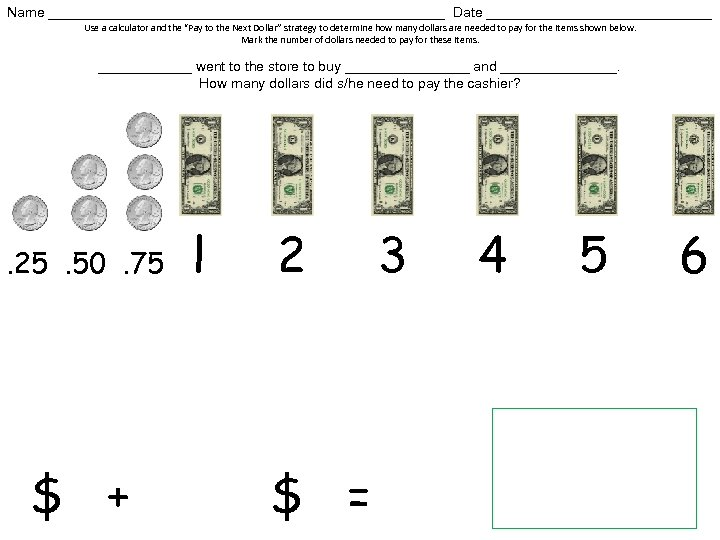Name __________________________ Date _______________ Use a calculator and the “Pay to the Next Dollar” strategy to determine how many dollars are needed to pay for the items shown below. Mark the number of dollars needed to pay for these items. ______ went to the store to buy ________ and ________. How many dollars did s/he need to pay the cashier? . 25. 50. 75 \$ + l 2 \$ = 3 4 5 6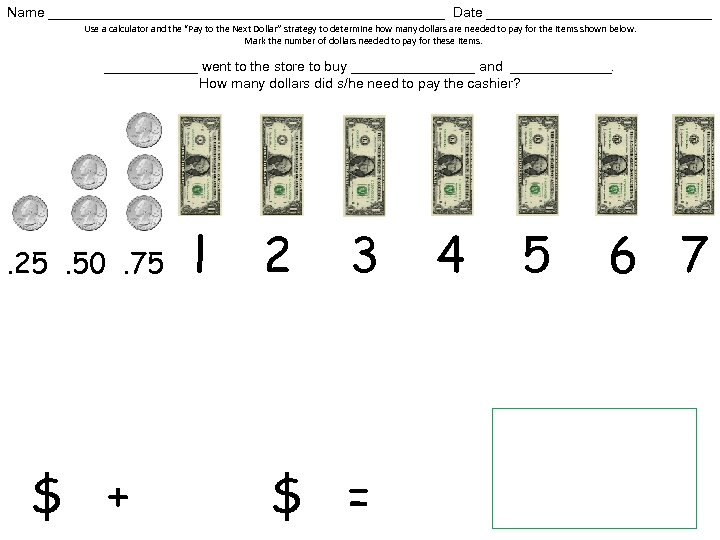Name __________________________ Date _______________ Use a calculator and the “Pay to the Next Dollar” strategy to determine how many dollars are needed to pay for the items shown below. Mark the number of dollars needed to pay for these items. ______ went to the store to buy ________ and _______. How many dollars did s/he need to pay the cashier? . 25. 50. 75 \$ + l 2 3 \$ = 4 5 6 7